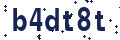## R Programming

• /
• Courses

Duration

22 Hours

Course Price

\$ 299.00

4.5 (23)

### 1. R programming Concept

• An Introduction to R
• Introduction to the R language
• Programming statistical graphics
• Programming with R
• Simulation
• Computational linear algebra
• Numerical optimization

### 2. More Advanced Concepts Of R Programming

• Vectors
• Matrices
• Factors
• Data Frames
• Lists
• R Graphics
• R built in functions and R packages.

• Naive Bayes
• Recommender
• K-means
• time series

### 5. Data Manipulation Techniques

• Data in R
• R and Databases
• Dates
• Factors
• Subscripting
• Character Manipulation
• Data Aggregation
• Reshaping Data

### 6. Statistical Applications using R programming

• Basics
• The R Environment
• Probability and distributions
• Descriptive statistics and graphics
• One- and two-sample tests
• Regression and correlation
• Analysis of variance and the Kruskal–Wallis test
• Tabular data
• Power and the computation of sample size
• Multiple Regression
• Linear models
• Logistic regression
• Survival analysis
• Rates and Poisson regression
• Nonlinear curve fitting

#### Trainer Profile